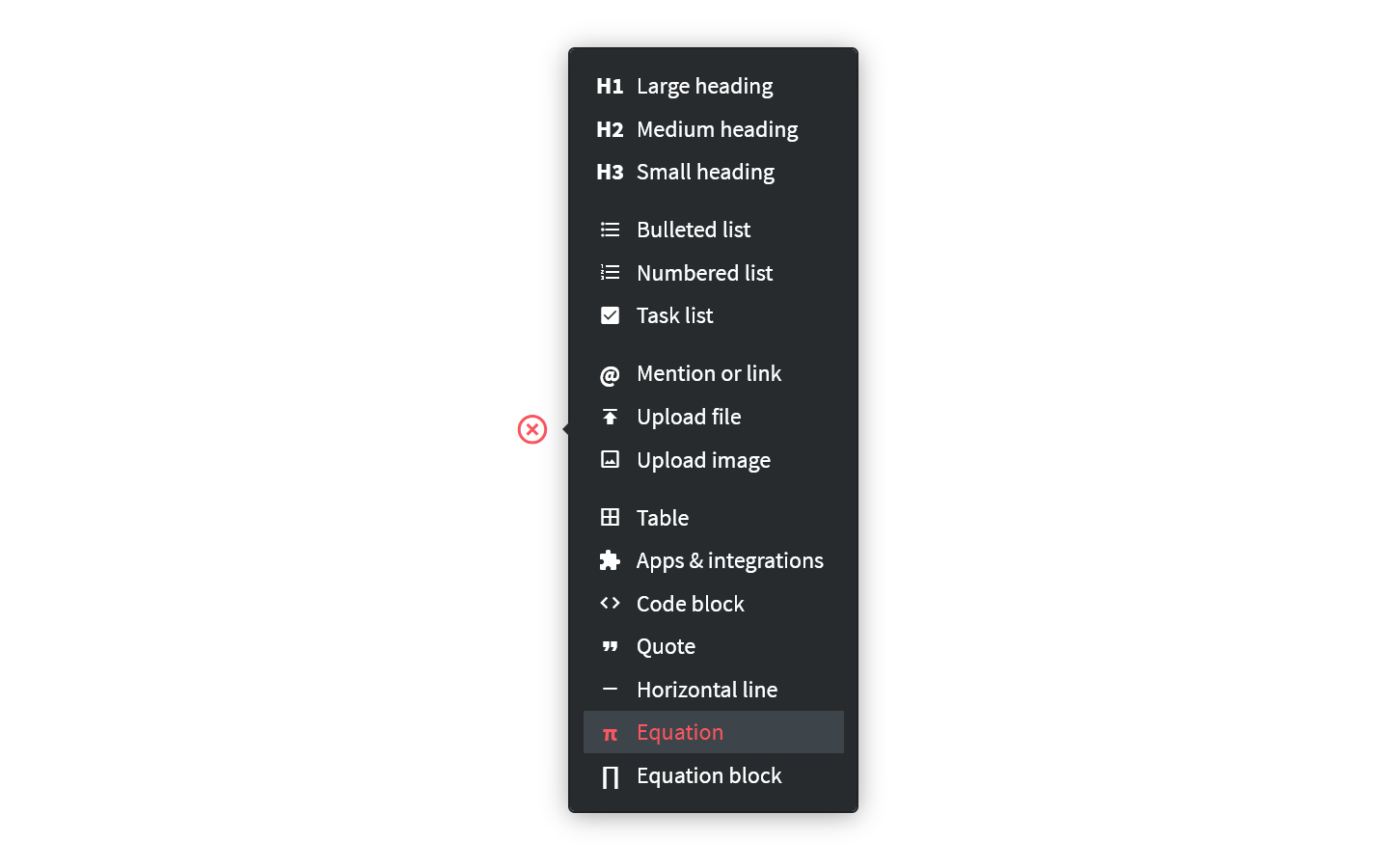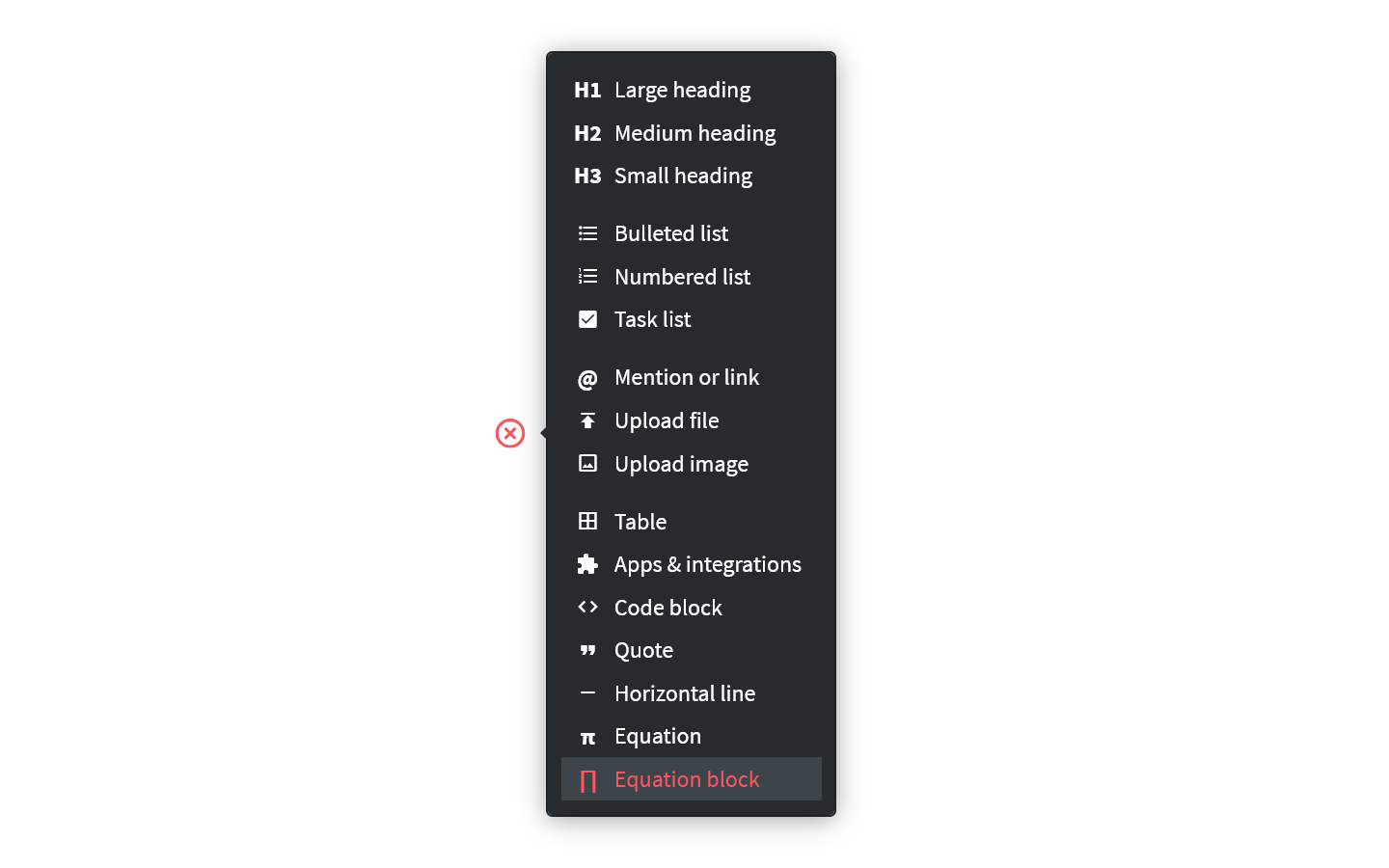# Use equations

Who can use this feature?

🔐 Only workspace editors can add and edit equations.

⭐️ Available on all plans.

Nuclino uses the KaTeX library to render mathematical equations and characters. KaTeX supports a large subset of LaTeX functions.

• Inline, e.g.

• As a dedicated content block, e.g.:

# To add an equation inline

## Using the slash command

1. Open the item in which you'd like to add an equation.

2. Type / inside a paragraph.

3. Select Equation (type to filter your options).4. Type or paste your equation.

5. Press Ctrl/Cmd + Enter to save the equation.1. Click on an empty line or create a new one.

2. Click on the insert button, the red + icon.

3. Select Equation.4. Type or paste your equation.

5. Press Ctrl/Cmd + Enter to save the equation.

## Using a keyboard shortcut

1. Type $$ inside a paragraph. 2. Type or paste your equation. 3. Type $$ at the end of the equation to render it.

For example, $$E=mc^2$$ becomes .

# To add an equation as a block

## Using the slash command

1. Open the item you'd like to add an equation block to.

2. Click on an empty line or create a new one.

3. Type /.

4. Select Equation block (type to filter your options).5. Type or paste your equation.

6. Press Ctrl/Cmd + Enter to save the equation.

1. Open the item you'd like to add an equation block to.

2. Click on an empty line or create a new one.

3. Click on the insert button, the red + icon.

4. Select Equation block.5. Type or paste your equation.

6. Press Ctrl/Cmd + Enter to save the equation.

# To edit an equation

1. Open the item which contains the equation you'd like to edit.

2. Click on the equation to select it.

3. Change the equation.

4. Press Ctrl/Cmd + Enter to save the equation.